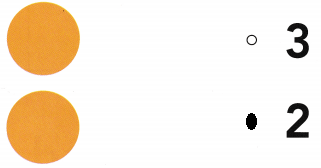# Texas Go Math Kindergarten Lesson 9.1 Answer Key Compose Numbers Up to 3

Refer to our Texas Go Math Kindergarten Answer Key Pdf to score good marks in the exams. Test yourself by practicing the problems from Texas Go Math Kindergarten Lesson 9.1 Answer Key Compose Numbers Up to 3.

## Texas Go Math Kindergarten Lesson 9.1 Answer Key Compose Numbers Up to 3

Explore

DIRECTIONS: Place red and yellow counters in the five frames as shown. There are 3 counters. Write the number of yellow counters. Write the number of red counters.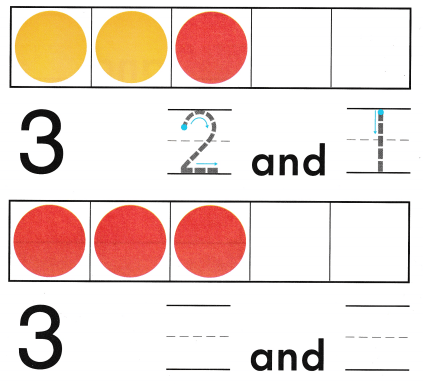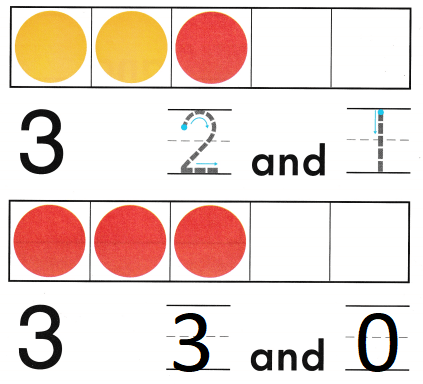Explanation:
The number of red counters are 3
the number of yellow counters are 0
Total number of counters 3

Share and Show

DIRECTIONS: Place counters in the five frames as shown. 1. There are 2 counters. Write the number of yellow counters. Write the number of red counters. 2. There are 3 counters. Write the number of yellow counters. Write the number of red counters.

Question 1.Explanation:
The number of red counters are 1
the number of yellow counters are 1
Total number of counters 2

Question 2.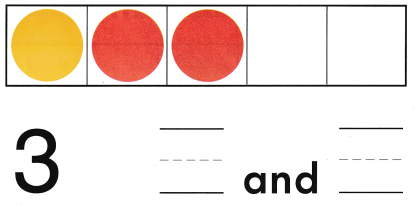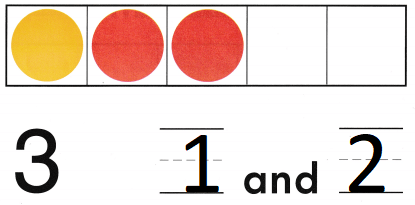Explanation:
The number of red counters are 2
the number of yellow counters are 1
Total number of counters 3

DIRECTIONS: 3-4. Place counters in the five frames as shown. Look at the number. Write the number of yellow counters. Write the number of red counters.

Question 3.Explanation:
The number of red counters are 0
the number of yellow counters are 2
Total number of counters 2

Question 4.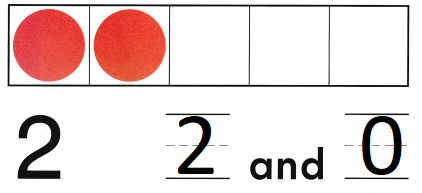Explanation:
The number of red counters are 2
the number of yellow counters are 0
Total number of counters 2

HOME ACTIVITY • Show your child a set of two objects. Have him or her add one more object to the set and tell how many there are now.Explanation:
Given set of balloons and then given one more balloon
2 + 1 = 3
so, total there are 3 balloons.

DIRECTIONS: 5. Sasha wants to have 3 counters. She has 2 yellow counters in the five frame. How many red counters does she need to have 3? Write the number of yellow counters. Draw and color the red counters. Write the number of red counters. 6. Choose the correct answer. How many dots in all?

Problem Solving

Question 5.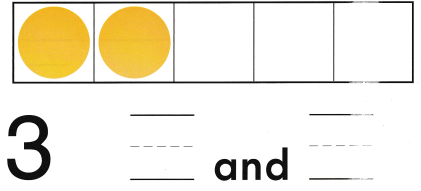Explanation:
Sasha wants to have 3 counters.
She has 2 yellow counters in the five frame.
1 red counters that she need to have 3
The number of red counters are 1
the number of yellow counters are 2
Total number of counters 3

Question 6.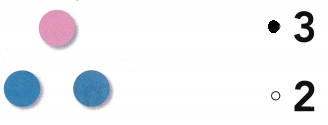Explanation:
There are 3 dots
2 dots are in blue color and 1 dot is in pink color.

### Texas Go Math Kindergarten Lesson 9.1 Homework and Practice Answer Key

DIRECTIONS: 1. There are 3 counters. Write the number of red counters. Write the number of yellow counters. 2. Look at the number. Write the number of yellow counters. Write the number of red counters.

Question 1.Explanation:
The number of red counters are 2
the number of yellow counters are 1
Total number of counters 3

Question 2.Explanation:
The number of red counters are 1
the number of yellow counters are 1
Total number of counters 2

DIRECTIONS: Choose the correct answer. 3-5. How many dots in all?

Question 3.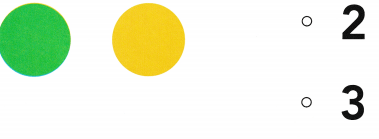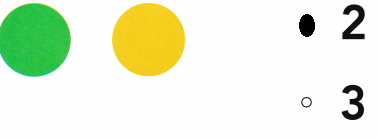Explanation:
There are 2 dots
one yellow dot and one green dot

Question 4.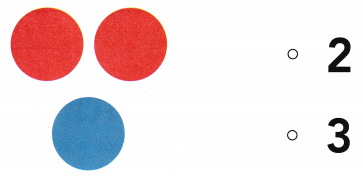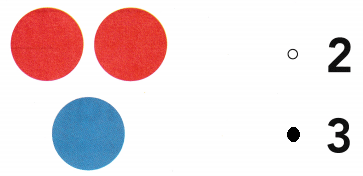Explanation:
There are 3 dots
2 red dots and 1 blue dot.

Question 5.